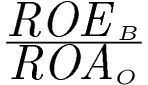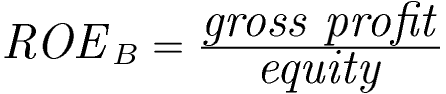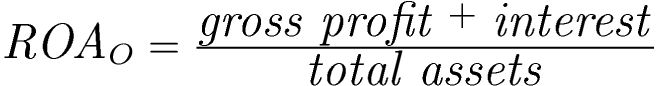Comprehensive software for monitoring and evaluation of the economic situation of the enterprise and early warning# Ratio of financial leverage effect

Method of calculationwhere:Ratio's description

The ratio is helpful in determining whether the company can achieve positive financial leverage effects. Positive financial leverage effects occur when the increase of debt level (i.e. new interest liabilities) result in an improvement of equity's profitability (ROEB). In other words, the ratio helps to assess whether new interest liabilities, taken with the current operating potential of the company (expressed by assets profitability ROAO) and current level of interest, allow to improve the profitability as seen by the owners (i.e. allow to increase the value of ROEB). The opposite effect is called a "financial club" (negative financial leverage effect) – it indicates the situation in which new interest liabilities lead to the worsening of equity's profitability, expressed by the decrease of the value of ROEB.

The ratio is very helpful in the managerial context – it allows to assess whether using external sources of financing is effective from the point of view of the owners; with the negative financial leverage effects ("financial club") the company should search for other sources of financing (e.g. by increasing the equity).

WARNING! The ratio uses the measures that are different from the traditional ROE i ROA; ROEB is the so called gross ROE, while ROAO is the so called operating ROA (the numerator represents simplified EBIT value – Earnings Before Interests and Taxes).

Ratio's interpretation

• If the ratio's value > 1 (meaning that ROE/ ROA>1), then positive financial leverage effects occur; in other words the increase of debt from interest will lead to the improvement of equity's profitability.
• If the ratio's value < 1 (meaning that ROEB / ROAO <1), then negative financial leverage effects occur; in other words the increase of debt from interest will lead to the deterioration of equity's profitability.
• When assessing the changes in ratio's value over time (over few periods):
• the increase of ratio's value is assessed positively – it indicates that the potential of the company to use positive financial leverage effects increases (in particular if the value increases much above the limit value of 1.0),
• the decrease of ratio's value is assessed negatively – it indicates that the potential of the company to use positive financial leverage effects decreases (in particular if the value becomes closer to the limit value of 1.0),

WARNING! If the values of ROEB and ROAO are not simultaneously positive then the value of the ratio cannot be reliably interpreted.

We use cookies to ensure you have the best experience on our website. If you continue to use this site, we will assume that you are happy with it.